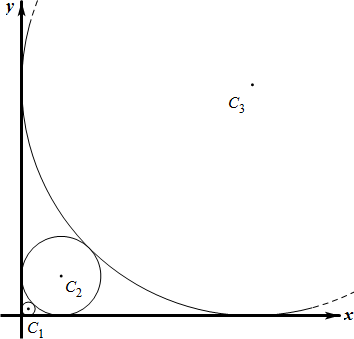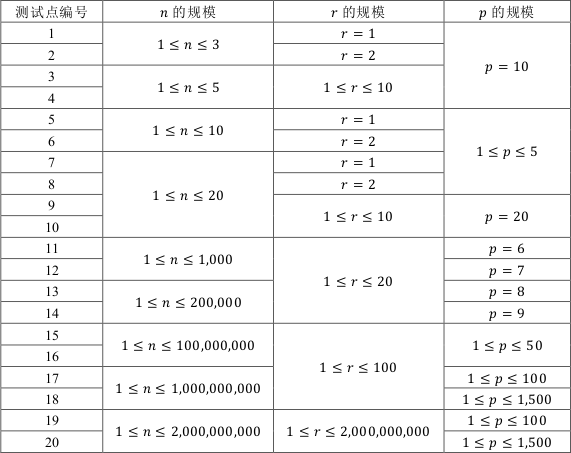# [研学] 质因数分解及素性判定

• ### 【1.1】记号与规定

1. 记 $$\displaystyle\mathbb{R}$$ 表示实数集，$$\displaystyle\mathbb{N}$$ 表示自然数集，$$P$$ 表示素数集。
2. 勒让德符号 $$\displaystyle\left( {\frac{n}{p}} \right)$$。设 $$\displaystyle p \in P,n \in \mathbb{N}$$。

• ### 【1.2】素数的一些性质

• $$P$$ 是无限集。
• 对于任意大于 $$1$$ 的自然数，它要么是个素数，要么可以分解为若干素数之积，并且在忽略顺序的情况下，这样的分解是唯一的。
• 小于 $$n$$ 的质数大约有 $$\ln n$$ 个。
• 一个合数 $$n$$ 最小的素因数因数一定小于 $$\sqrt n$$。
• 费马小定理：设 $$p$$ 是大于 $$2$$ 的素数，则对于任意正整数 $$n$$ 均有 $$\displaystyle \begin{array}{*{20}{c}}{{n^{p – 1}} \equiv 1}&{(\bmod p)}\end{array}$$。
• Mertens’ second theorem

# YZOJ P4621 [CSP-S 2019 四校联训 Round 1]生日悖论

• ### 数据规模与约定

$$1 \leq N \leq 10^{18}$$，$$2 \leq k \leq 10^{18}$$ 。

# YZOJ P4587 斐波那契数列

• ### 样例输出

Source： BZOJ 5104…

…

…

# YZOJ P2163 [THUSC2015]解密运算

• ### 数据规模与约定

$$N,M \leq 200000$$ 。

…

# YZOJ P2697 画圆

• ### 题目描述

$$Alkri$$ 想在平面直角坐标系的第一象限中依次画 $$n$$ 个与两坐标轴均相切的圆，其中，第 $$1$$ 个圆的半径为 $$r$$，之后的每个圆都比上一个圆大，且与上一个圆相切，也就是说，对所有整数 $$2 \leq i \leq n$$，第 $$i$$ 个圆的半径大于第 $$i-1$$ 个圆的半径且与第 $$i-1$$个圆相切。• ### 数据规模与约定…